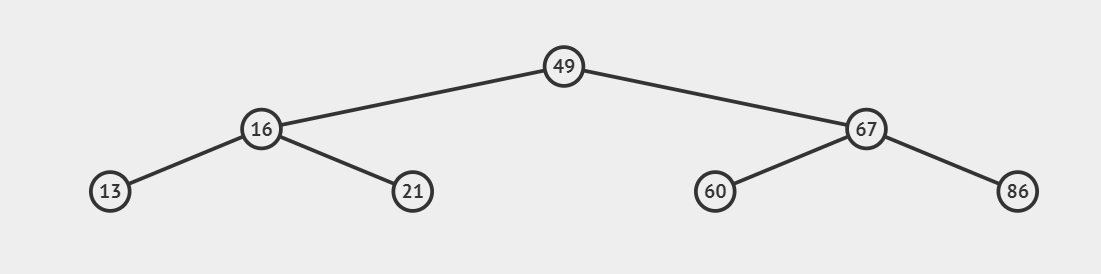# Maximum Absolute difference between any two levels of binary Tree

## Introduction

The problem states that we are given a binary tree, and we need to calculate the maximum absolute difference between any two levels of a binary tree. The value of nodes of the binary tree can be negative, and the height of the binary tree is greater than two.

### Revisiting Binary Tree

A binary tree is a data structure where each node can have a maximum number of two children. These child nodes of the binary tree are known as the left child and right child.

### Prerequisites

To Find the maximum absolute difference between any two levels, a evel order traversal of a binary tree is necessary. To know about you can refer this

### Sample Examples

Input: Given the binary tree, Find the maximum absolute difference between any two levels.``````Output: The maximum absolute difference between any two levels is : 131
Explanation:
The sum of level 0 = 49
The Sum of level 1 = 16 + 67 = 83
The Sum of level 2 = 13 + 21 + 60 + 86 = 180 ``````

## Approach

To find the maximum absolute difference between any two levels, we need to find only the minimum and maximum sum levels. Their difference will always give us the maximum absolute difference between any two levels of the binary tree. This can be found by using the level order traversal of the binary tree. At each level, we will check for maximum and minimum sums.

### Algorithm

1. Traverse the binary tree in a level order fashion using the queue data structure.
2. We will keep the two variables maxSum and minSum to keep the track of maximum and minimum sum of levels of a binary tree.
3. We will process the nodes of the binary tree in level order fashion and find the sum of each level, and then compare it with maxSum and minSum.
4. Then return the absolute difference between maxSum and minSum.

### Implementation in C++

``````// C++ program to find the maximum
// absolute difference of level
// sum in Binary Tree
#include <bits/stdc++.h>
using namespace std;

// Class containing left and
// right child of current
// node and key value
class BinaryTreeNode
{
public:
int data;
BinaryTreeNode *left, *right;
BinaryTreeNode(int data){
this->data = data;
left = NULL;
right = NULL;
}
};

// Function to find the
// maximum absolute difference of level
// sum in binary tree
// using level order traversal
int MaximumAbsoluteDifference(BinaryTreeNode *root)
{

// Initialize value of maximum
// and minimum level sum
int maxsum = INT_MIN;
int minsum = INT_MAX;

queue<BinaryTreeNode*> q;
q.push(root);

// Do Level order traBinaryTreeNodeversal
// keeping track of number
// of nodes at every level.
while (!q.empty())
{

// Get the size of queue when
// the level order traversal
// for one level finishes
int s = q.size();

// Iterate for all the nodes in
// the queue currently
int sum = 0;

for(int i = 0; i < s; i++)
{

// Dequeue an node from queue
BinaryTreeNode*t = q.front();
q.pop();

// Add this node's value to
// the current sum.
sum += t->data;

// Enqueue left and
// right children of
// dequeued node
if (t->left != NULL)
q.push(t->left);

if (t->right != NULL)
q.push(t->right);
}

// Update the maximum
// level sum value
maxsum = max(maxsum, sum);

// Update the minimum
// level sum value
minsum = min(minsum, sum);
}

// return the maximum absolute difference
// of level sum
return abs(maxsum - minsum);
}

// Driver code
int main()
{
BinaryTreeNode *root = new BinaryTreeNode(49);
root->left = new BinaryTreeNode(16);
root->right = new BinaryTreeNode(67);
root->left->left = new BinaryTreeNode(13);
root->left->right = new BinaryTreeNode(21);
root->right->left = new BinaryTreeNode(60);
root->right->right = new BinaryTreeNode(86);

cout << "The maximum absolute difference between any two levels is " << MaximumAbsoluteDifference(root) << endl;
}``````

Output:

``The maximum absolute difference between any two levels is 131. ``

#### Complexity Analysis

Time Complexity: O(N)

Explanation: O(N) because we are traveling only the binary tree once for level order traversal.

Space Complexity: O(N)

Explanation: We are using queue data structure for level order traversal which is taking almost O(N) space.

Q1. What is the difference between a binary tree and a binary search tree?

Ans. A tree that has a maximum of 2 children is called a binary tree, whereas a binary search tree is a particular binary tree having the following properties.

Keys in the left subtree are always smaller than the root’s node, whereas keys in the right subtree are greater than the root’s node.

Q2. What is inorder traversal ?

Ans. In inorder traversal, we first traverse the left subtree, then visit the root and then traverse the right subtree.

Q3. Which data structure is used in level order traversal and inorder traversal?

Ans. Level Order Traversal = queue

Inorder Traversal = Stack

## Key takeaways

This article discussed getting the maximum absolute difference between any two levels of a binary tree. We hope you understand the problem and solution properly. Now you can try the more similar questions on BST.

If you are a beginner, interested in coding, and want to learn DSA, you can look for our guided path for DSA, which is free!